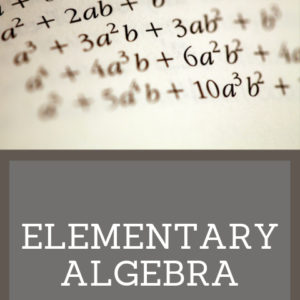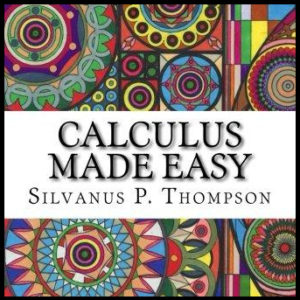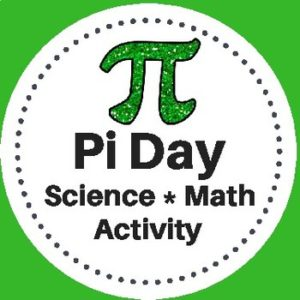Showing all 13 results

•\$10.00

Elementary Algebra is designed to meet the scope and sequence requirements of a a full year of elementary algebra. Each topic builds upon previously developed material to demonstrate the cohesiveness and structure of mathematics. Topics studied:

Arithmetic Review
Real Numbers
Algebraic Expressions & Equations
Linear Equations & Inequalities
Polynomials
Graphing Linear Equations & Inequalities
Rational Expressions
Roots, Radicals and Square Root Equations
Systems of Linear Equations

•\$3.00

Calculus Made Easy is a book on infinitesimal calculus originally published in 1910 by Silvanus P. Thompson, considered a classic and elegant introduction to the subject.

•\$3.00

This Pi Day Activity has been designed for Science and Math classrooms. Students will use measuring tools to determine the radius, diameter, and circumference of various circular objects. They will then use the data that they collect to calculate pi.

*This activity gives students the opportunity to clearly understand the concept of the number pi and practice math skills through a hands-on activity with a variety of circular objects.

•\$3.50

Written in 1895, this text includes over 1000 exercises for practice. Topics covered include operations, factoring, fractions, complex fractions, solving equations, and solving simultaneous equations. Answers are provided at the end of the book.

•\$9.99

This algebra textbook teaches students the following:

• Pre-Algebra
• Solving Linear Equations
• Graphing
• Inequalities
• Systems of Equations
• Polynomials
• Factoring
• Rational Expressions
• Functions

•\$3.00
Here are 3 colorful “Geometry Gem” posters to display in your classroom or to place in a math, learning center as a reference.
See description below for a list of what is included!
•\$5.00

121 Math Posters – Each displaying a math term and explanation!

Designed for 4th – 12th Grades

•\$19.99

Complete High School Geometry course

•\$14.99

•\$5.00

Here are 250 word problems with answer keys. There are 25 sets, each with 10 problems each.

•\$4.00

This 123 page math resource will help students who are learning (or struggling to understand) algebra better by giving detailed explanations of related terminology and concepts. This book as been categorized as curriculum because of the detailed explanations of the topics. It however, is for instructional use only and does not include student problems.

(See description below for covered topics)

•\$9.99

A step by step Geometry curriculum to teach students with ease. Students will learn about angles, lines, triangles, polygons,quadrilaterals, prisms, coordinate geometry, slope and more!

•\$9.99

Help students prepare for the Math SAT with this resource. This 254 page workbook will help students with…

• – Techniques and Strategies
• – Numbers & Operations Review
• – Algebra Review
• – Geometry Review
• – Problem Solving
• – 3 Practice Tests – with answer key and explanations
• – Math glossary